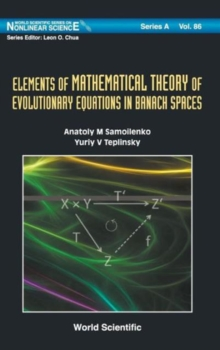Supporting your high street Find out how »
• My Account# Elements Of Mathematical Theory Of Evolutionary Equations In Banach Spaces Hardback

## Part of the World Scientific Series on Nonlinear Science Series A series

#### Description

Evolutionary equations are studied in abstract Banach spaces and in spaces of bounded number sequences.

For linear and nonlinear difference equations, which are defined on finite-dimensional and infinite-dimensional tori, the problem of reducibility is solved, in particular, in neighborhoods of their invariant sets, and the basics for a theory of invariant tori and bounded semi-invariant manifolds are established.

Also considered are the questions on existence and approximate construction of periodic solutions for difference equations in infinite-dimensional spaces and the problem of extendibility of the solutions in degenerate cases.

For nonlinear differential equations in spaces of bounded number sequences, new results are obtained in the theory of countable-point boundary-value problems.The book contains new mathematical results that will be useful towards advances in nonlinear mechanics and theoretical physics.

#### Information

• Format: Hardback
• Pages: 408 pages
• Publisher: World Scientific Publishing Co Pte Ltd
• Publication Date:
• Category: Functional analysis & transforms
• ISBN: 9789814434829

£116.00

£100.69£130.00

£112.55

£195.00

£156.00

£84.00

£69.05

£130.00